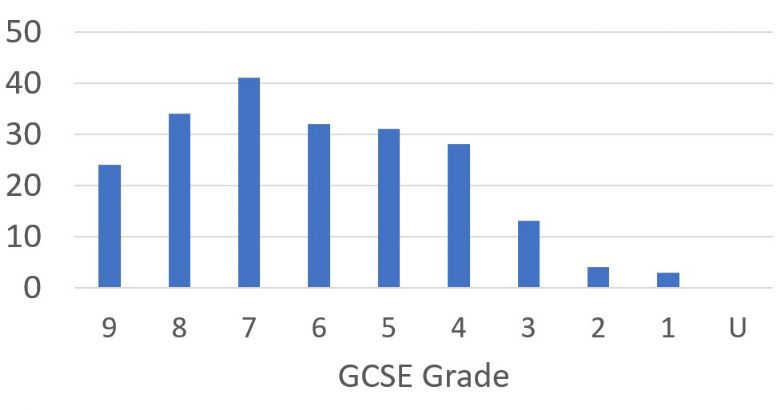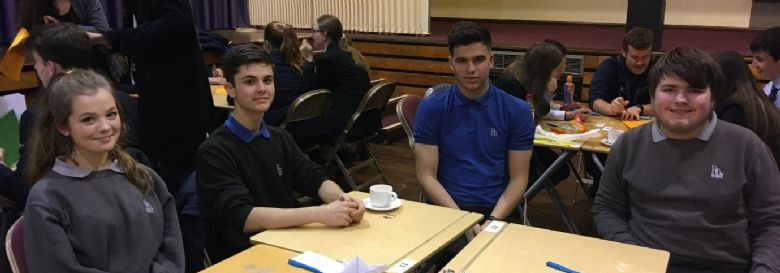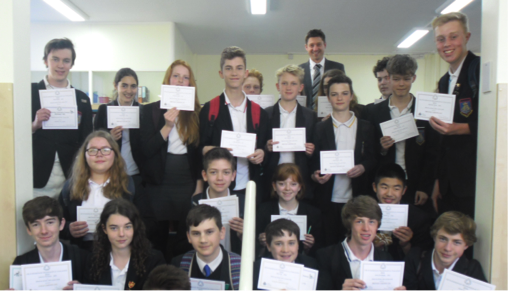# Mathematics

### Key to Curriculum maps

 Basic numeracy Geometry Sequences Probability Ratios, Proportion, Fractions, Decimals, Percentages Statistics Algebra

### Curriculum map Key Stage 3

 YEAR 7 YEAR 8 YEAR 9 September Numeracy toolbox Standard form Indices Roots Standard form Error intervals Calculating with negatives October Index laws, Factorising and changing the subject Constructing Bisectors & Loci Expanding & Factorising quadratics November Factors and Multiples Enlargement & Bearings Direct and inverse Proportion Ordering integers, decimals, fractions Probability language and notation Fibonacci & Quadratic sequences December Multiplying & dividing decimals Converting FDP Time series graphs, Frequency polygons & Scatter graphs Constructing & Labelling January Introduction to alegra Ratios and proportion Circle Sectors and cylinders Angle rules and 2D shapes Linear sequences Solving Inequalities February Parallel lines & polygons Similarity and Congruent Triangles March Multiplier method Straight lines and Quadratic graphs Units of length, mass, volume, Ratios Solving equations Simultaneous equations April Solving equations Circles Probability May Straight line graphs Transformations Percentages Probability diagrams and trees Percentages June Coordinates & Transformations Statistics Pythagoras Measuring & Presenting data Trigonometry July

### Curriculum map GCSE (higher level)

 September Year 10 Year 11 Calculations, checking, rounding, product rule Further Trigonometry Indices (including roots and reciprocals) Graphs of trigonometric functions Factors, multiples & primes Collecting data (including sampling & capture-recapture) Standard form and surds Cumulative frequency, box plots and histograms October Algebra: The basics Sketching algebraic graphs Solving linear equations (including setting up and rearranging formulae) Circle Theorems Test and Review Circle Geometry November Sequences Mocks Averages and range Representing and interpreting data Direct and inverse proportion (both numerical and algebraic approach) Scatter graphs Fractions December Gradient and area under (non-linear) graphs and graph transformations Percentages (taught in Y9) Test and Review January Ratio Angles (including polygons and parallel lines) Vectors Pythagoras' Theorem and Trigonometry (taught in Y9) Similarity and congruence, 2D and 3D February Straight line graphs Revision Test and Review Real straight line graphs and coordinate geometry March Quadratic, cubic, reciprocal, exponential and circle graphs Perimeter, area and 3D forms Circles, cylinders, cones and spheres April Exams May Accuracy and bounds Exams Transformations Constructions, loci and bearings June Solving quadratic equations (including linear and non linear simultaneous equations) algebraically Inequalities (linear and quadratic)

### Curriculum map GCSE (Foundation)

 September 10 11 integers & place value Pythag revision and trigonometry multiplicative reasoning decimals probability 1 indices, powers & roots probability 2 October factors, multiples & primes constructions, loci & bearings algebra, the basics quadratic equations, expanding & factorising November Mocks expanding & factorising single brackets expressions & substitution into formulae December tables plans, elevations & nets Charts and Graphs quadratic equations, graphs January Pie Charts circles, cylinders, cones & spheres Scatter Graphs fractions & reciprocals Fractions indices & standard form revision similarity & congruence in 2D Fractions, decimals, percentages vectors February rearranging equations, graphs of cubic & reciprocal functions Revision: Percentages (taught in 9) Revision Stats and sampling March The averages polygons & parallel lines interior & exterior angles of polygons equations April inequalities sequences Exams perimeter & area 3D forms & volume June straight line graphs real life graphs

### Curriculum map A level mathematics

 Pure- 5 hours a fortnight Mechanics- 2 hours a fortnight Statistics- 2 hours a fortnight
 Y12 Y13 Sept Indices and Surds Proof Quadratics Functions and mappings Definitions and Large Data Set Correlation Measures of location Modelling Moments Constant Acceleration Oct Equations and Inequalities Binomial expansion Graphs and transformations Radians Measures of spread Probability Constant Acceleration Forces and friction Nov Coordinate Geometry Trigonometric functions Circles Trigonometry and modelling Representing data Probability Forces Projectiles Dec Algebraic Methods Parametric equations Correlation Normal Distribution Forces Projectiles Jan Binomial expansion Mocks Trigonometric ratios Trigonometric identities Vectors (in mechanics lessons) Differentiation Probability Application of forces Distributions Normal Distribution Feb Mocks Differentiation Differentiation Numerical methods Hypothesis tests Normal Distribution Variable acceleration Application of forces Mar Differentiation Integration Integration Vectors Hypothesis tests Revision of Y12 content Variable acceleration Vectors in kinematics Apr Exponentials and Logarithms Revision and past papers Revision of Y12 content Revision and past papers Revision of Y12 content Revision and past papers May Revision of Y12 content EXAMS

### Curriculum maps Further Maths A level

 Core Pure- 5 hours a fortnight Mechanics- 2 hours a fortnight Statistics- 2 hours a fortnight
 Y12 Y13 Sept Complex Numbers Series Argand Diagrams Methods in calculus Discrete Random Variables Negative Binomial Distribution Momentum and impulse Momentum and impulse Work, energy and power Revision of Y12 collisions Oct Matrices Volumes of revolution Discrete Random Variables Hypothesis Testing- Geometric Work, energy and power Elastic collisions in 2 dimensions Nov Linear Transformations Polar coordinates Poisson Distribution Probability Generating Functions Elastic Collisions Elastic collisions in 2 dimensions Dec Series Hyperbolic Functions Proof by induction Probability Generating Functions Poisson Distribution Revision of Y12 Energy Elastic Collisions Revision for mocks Jan Vectors Mocks Hypothesis Testing- Poisson Methods in differential equations Revision Central Limit Theorem Mocks Elastic springs (Hooke's law) Feb Volumes of revolution Modelling with differential equations Chi-Squared - discrete distributions Central Limit Theorem Revision and past papers Elastic springs (Hooke's law) Mar Revision and past papers Revision and past papers Chi-Squared - discrete distributions Quality of Tests Revision and past papers Revision and past papers Apr Revision and past papers Revision and past papers Chi-Squared - contingency tables Quality of Tests May A2 Complex Numbers REVISION AND EXAMS

### How is Mathematics organised at Balcarras School?

Year 7 (Y7) is split into two bands with each band containing 3 or 4 tutor groups. For the first half term they are taught as a tutor group. After the October half-term they are put into sets based on their raw KS2 results. If a pupil has not taken KS2 exams or their school does not provide the raw scores, we will use the numeracy test that all pupils sit at the end of the first half term to place them.

Being placed in a lower set does not mean that a pupil is not good at mathematics; it merely reflects their relative position on entry in an intake of nearly 200 pupils. Some pupils and parents find the initial setting to be a worry, especially if a child was one of the best mathematicians in a small primary school but we have found that the only fair way to allocate starting sets is to use the KS2 raw score and rank pupils. It is our aim to stretch and challenge pupils in all sets and at all abilities.

Again this year, pupils who have started at Balcarras in the bottom set in Y7 have achieved grade 8 at GCSE in Y11.

During Y7, changes are made between sets if it is clear that a student is wrongly placed.

All Y7 pupils take a common examination and this, together with performance in class work and homework throughout the year, forms the basis of set allocation for Y8 within two parallel half-year bands.In Y9 pupils are placed in sets 1-9 as a full year group. In Y10 and Y11 we go back to two parallel half-year bands. Within each band pupils are setted for mathematics based on their exam and class work.We always enter students into the highest level paper that we believe will maximize their grade in external examinations

## Key Stage 3

Follow the kangaroo maths Key Stage 3 Framework for Teaching Mathematics, with a strong mastery approach. In accordance with the National Strategy we aim to develop both mental and written mathematical skills.

The scheme mirrors the requirements of the national curriculum and includes number and algebra, geometry and measures, statistics together with mathematical processes and applications.

Being in smaller set groupings, aided by Teaching Assistants where appropriate, supports the least able.

We set and internally assess KS3 SAT examinations to determine setting for Y10.

## GCSE

All students follow a course of study that can lead to a GCSE examination in mathematics. We follow the Edexcel specification and pupils sit three formal examinations at the end of the course. There is no coursework in mathematics. The examinations include elements of ‘functional skills’ and the final grade will be between 9 and 1, with foundation students able to achieve a maximum of a grade 5.

Results are consistently well above the national average and amongst the highest in the county.

In June 2019, 90.5% of the students in Y11 achieved an 9 - 4 grade in mathematics with 47.1% of pupils gaining a grade 7 or better and 11.4% achieving a grade 9.

 2019 90.5% 9-4 in Maths 2018 89.7% 2017 94.8% 2016 86.1% A*-C in Maths 2015 91.3% 2014 90.5% 2013 93.6% 2012 95% 2011 90%

### GCSE Mathematics 2019## A level

Advanced level mathematics is growing in popularity and is relevant to most career choices. We offer units in Pure Maths, Mechanics, Statistics and Decision Maths. Balcarras School has an enviable reputation for A Level success.

In 2019, at A Level, 21 students achieved an A* grade and over 80% achieved at least a grade A. This gave the department an ALPS grade 1 making it the best mathematics department in the country (including grammar schools and independent schools). In A Level Further maths 85% achieved at least a grade A. Many of these students went on to study maths at some of the best universities in the country.

• Mathematics was the most applied for subject in the sixth form in 2017, 2018 and 2019
• Balcarras is one of the few schools in the county that offers both A level Mathematics and A level Further Mathematics

### What equipment will be needed?

In maths lessons you will need to have a pen, pencil and rubber together with mathematical drawing instruments (e.g. compass, ruler and protractor).

We expect all students to bring and use a scientific calculator and this is essential for two of the examinations papers. The school has mathematical equipment and calculators for sale and these can be purchased from any maths teacher.

• Items of equipment will be on sale during the new Y7 induction meeting in June
• A fixed price pack includes a calculator and all the equipment needed

### Does the school take part in any mathematical competitions?

Yes. We regularly enter students for the UKMT Mathematical Challenges at Junior, Intermediate and Senior Levels as well as the regional Team Mathematics Challenges.We run a maths club for Y11, 12 and 13 pupils every Thursday lunchtime which prepares students for the Maths Challenges and also for STEP/ MAT exams prior to University applications.